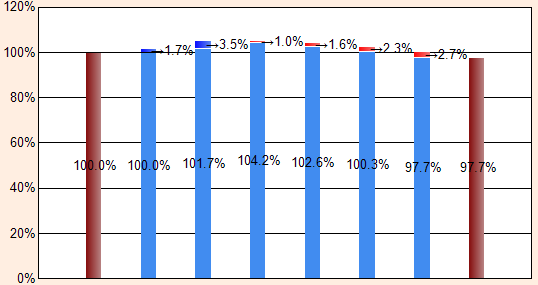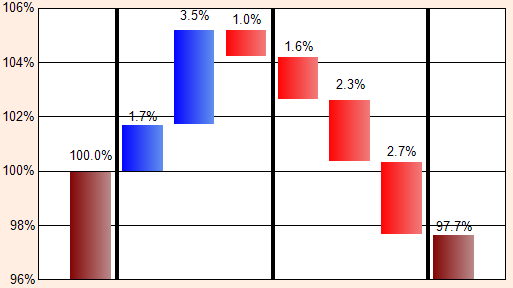## 2012-11-07

### SSRS - Stacked Column Chart - Place label outside column

When I create a normal Column Chart, go to Series\Properties\Label\Position, and select which position I want to place the label. Once I create Stacked Column Chart, this attribute seems doesn't work, neither does the SmartLabels section, after I searched relevant articles about this issue, there is a way to enable SmartLabels function by shrinking column width: Series\Properties\CustomeAttributes\PointWidth.

But that would not be an ideal solution if I don't want to narrow column width, then I made another research focusing on system built-in options (#VALX, #VALY, etc.) of label data, I found the way to format built-in options, right-click the label, and go to Series Label Properties\Label data, click expression button, type script as follows:
="            →#VALY{##0.0%}"
, the number of space ahead the arrow depend on column width, see the result:, for more formats please refer to: http://msdn.microsoft.com/en-us/library/0c899ak8.aspx. If you want to make it dynamic, you can create a function, then pass column width to that function, run loops to control space string and cooperate with column width.

There is another sample of Stacked Column Chart below. There are four stacked value fields come from stored procedure.
1. stacked_field_1: Maroon
2. stacked_field_2: Light Blue
2. stacked_field_3: Red
2. stacked_field_4: BlueThere are two challenges in this chart:
1. Place label on the top of series.
2. Automatically adjust Y axis value (Minimum, Maximum and Interval), otherwise, the result will similar to graph 1.

This kind of chart also can be generated in Crystal Reports, and it's very easy to configure label's position, but it can't use dynamic/automatic Y axis in the chart.

The X axis field has been put into Row Groups of the chart, right-click the group, and go to Group Properties\Variables, click add variable and type script:

1. Max
=IIF(MAX(Fields!stacked_field_2.Value + Fields!stacked_field_4.Value)<1, 1, MAX(Fields!stacked_field_2.Value + Fields!stacked_field_4.Value))
2. Interval (I control number of interval equal to 5, prevent too many grid lines)
=ROUND( (Variables!Max.Value - Min(Fields!stacked_field_2.Value))*100/5 )
3. Maximum
=100 + ( CEILING ( (Variables!Max.Value*100 - 100 + 0.1) / Variables!Interval.Value ) * Variables!Interval.Value )
4. Minimum
=100 - ( CEILING ( (100 - Min(Fields!inner_vals.Value)*100 + 0.2) / Variables!Interval.Value) * Variables!Interval.Value )
Right-click Y axis, go to Vertical Axis Properties, and click expression of Minimum and type script:
= Variables!Minimum.Value / 100
, same concept of settings for Maximum and Interval.

I found that label data can be formatted, then I started to think how to dynamically control change line character for each series. In the beginning, I just considered area value of each series, then I found the other elements are more important than area value: density of chart and fontsize of label, then I created a function:
Public Function SetLabelPosition(Item As String, AreaValue As Decimal, MaximumValue As Int32, MinimumValue As Int32, IntervalValue As Int32, FontSize As Decimal) As String
Dim change_line As String
Dim increase_time As Int32
Dim fs = 0.25 * FontSize / 10
Dim density As Decimal

change_line = ""
increase_time = 0
density = ((MaximumValue - MinimumValue)/IntervalValue)/5

If Item <> "stacked_field_1" Then
increase_time = Round(AreaValue/fs/IntervalValue/density) + 1
Else
increase_time = Round(AreaValue/fs/IntervalValue/density)
End If

'change_line += "\n IT: " + increase_time.ToString() + "\n"

If increase_time = 0 Then
increase_time = 1
Else If increase_time > 10 Then
increase_time = increase_time - 1
End If

For i As Int32 = 1 To increase_time
change_line += "\n " '+ i.ToString()
Next

'change_line += "Area: " + AreaValue.ToString() + "\n"
'change_line += "Max: " + MaximumValue.ToString()  + "\n"
'change_line += "Min: " + MinimumValue.ToString()  + "\n"
'change_line += "Inteval: " + IntervalValue.ToString()  + "\n"

Return change_line
End Function
Right-click the label of stacked_field_1, and click expression of Label data and type:
="#VALY{##0.0%}\n" +
Code.SetLabelPosition
(
"stacked_field_1",
SUM(Fields!outer_vals.Value)*100 - Variables!Minimum.Value,
Variables!Maximum.Value,
Variables!Minimum.Value,
Variables!Interval.Value,
10
)
, same configuration method for the other stacked fields.